## Figures index

#### Mohammadamin Azimi, Azlan Bin Adnan, Mohd Hanim Osman, Abdul Rahman Bin Mohd Sam, Iman Faridmehr, Reza Hodjati

American Journal of Civil Engineering and Architecture. 2014, 2(1), 42-52 doi:10.12691/ajcea-2-1-5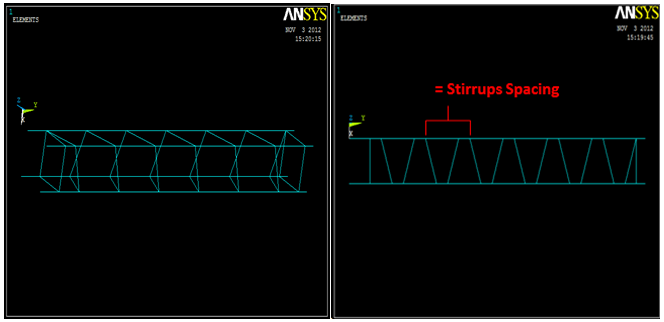• Figure 1. Single Square Spring Shear Resistant System (SSSSRS)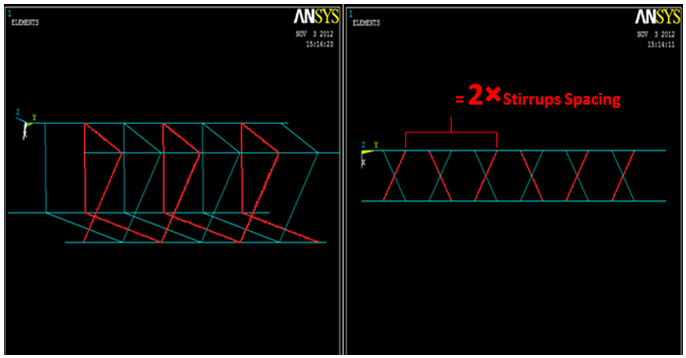• Figure 2. Double Square Spring Shear Resistant System (DSSSRS)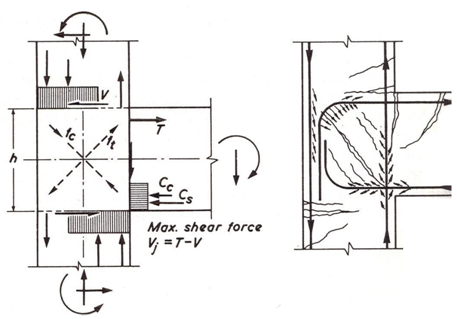• Figure 3. Force action in connections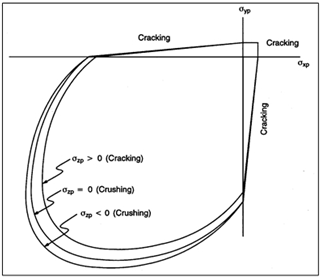• Figure 4. 3-D failure surface for concrete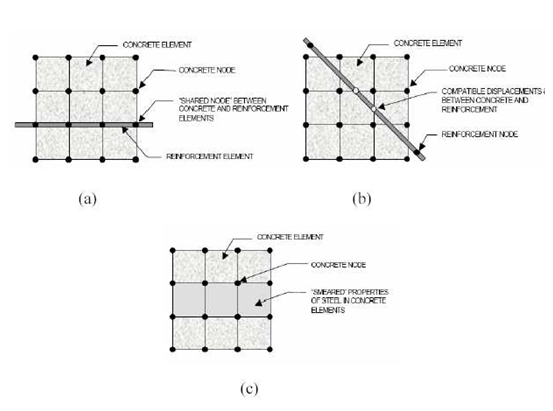• Figure 5. Models for Reinforcement in Reinforced Concrete [6,9]: (a) discrete; (b) embedded; and (c) smeared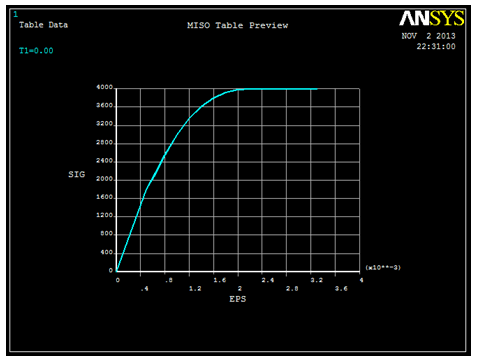• Figure 6. Uniaxial Stress-Strain Curve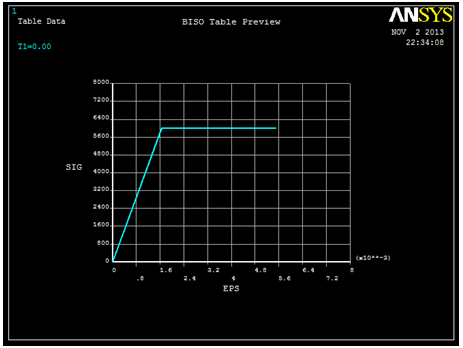• Figure 7. Idealized Stress-Strain Curve of Reinforcing Steel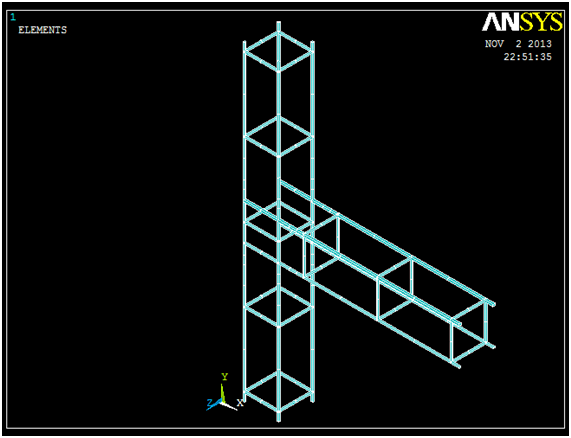• Figure 8a. Typical View of ANSYS Model for conventional design according to Euro code for Low Ductility Classes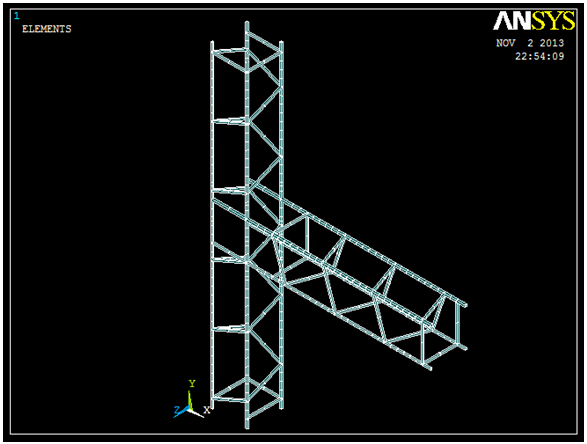• Figure 8b. Typical View of ANSYS Model for Single Square Spring Shear Resistant System (SSSSRS)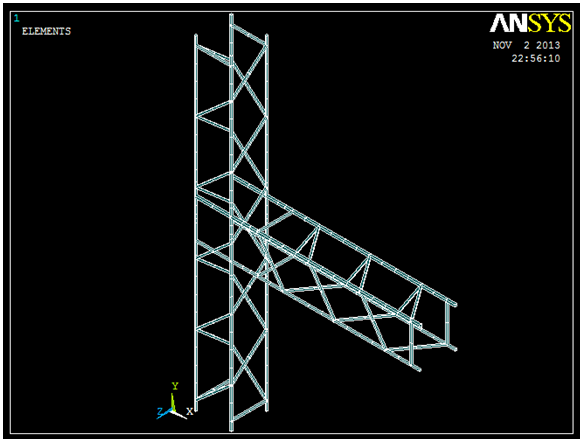• Figure 8c. Typical View of ANSYS Model for Double Square Spring Shear Resistant System (DSSSRS)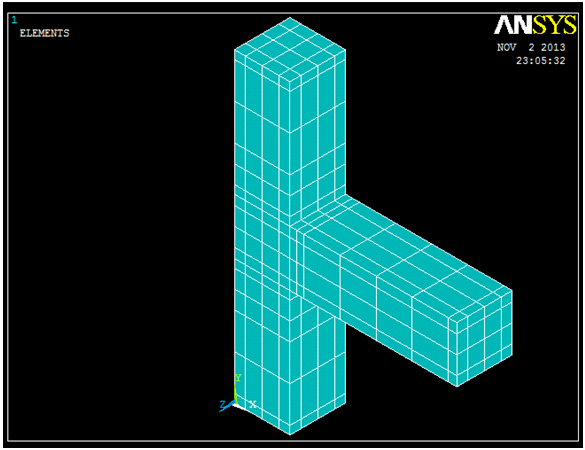• Figure 9a. Typical meshed Control Specimen conventional design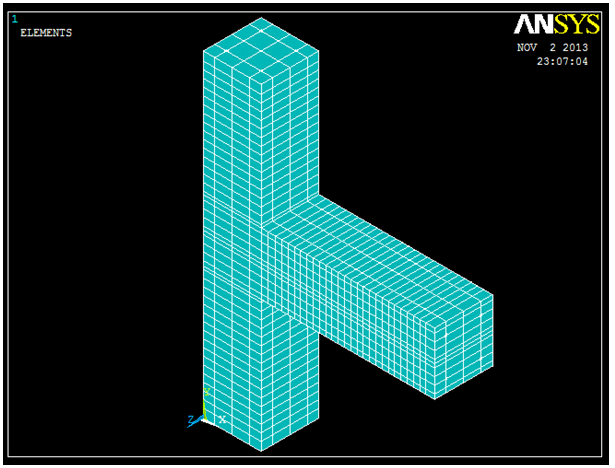• Figure 9b. Typical meshed Single Square Spring Shear Resistant System (SSSSRS) Specimen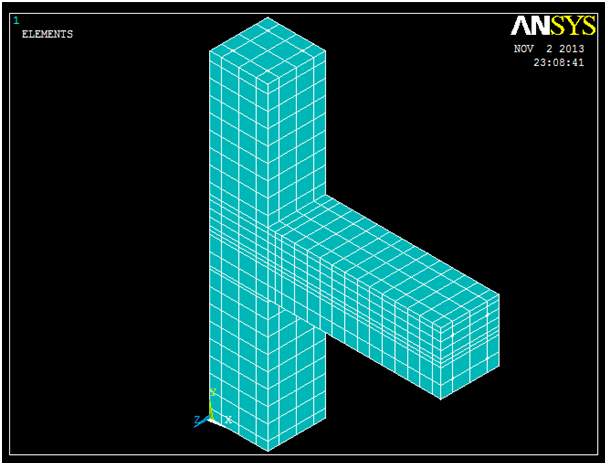• Figure 9c. Typical meshed Double Square Spring Shear Resistant System (DSSSRS) Specimen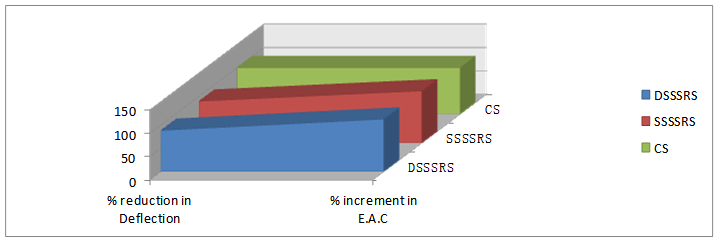• Figure 10. Comparison between Deflection and Energy Absorption Capacity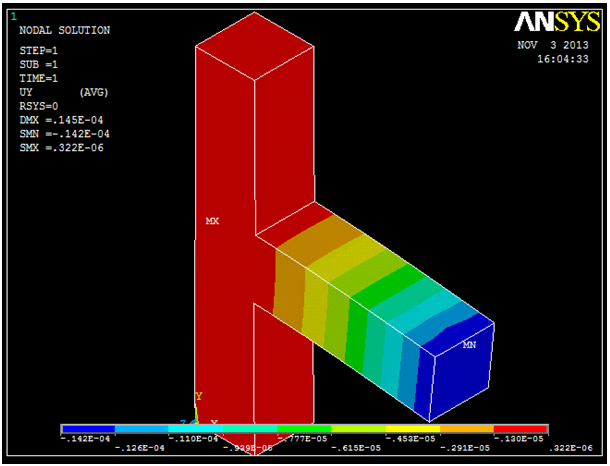• Figure 11a. Typical View of Deflected Control Specimen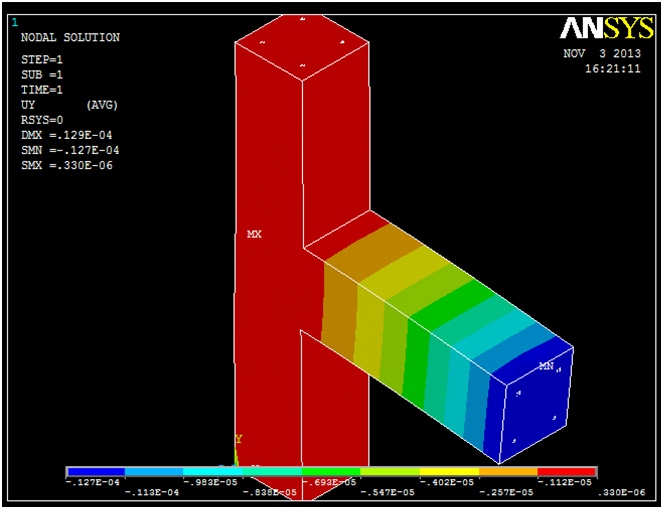• Figure 11b. Typical View of Deflected Specimen with Single Square Spring Shear Resistant System (SSSSRS)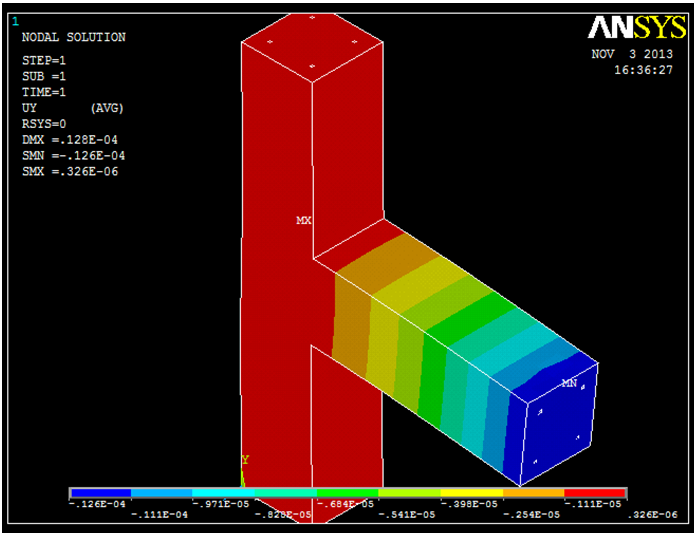• Figure 11c. Typical View of Deflected Specimen with Double Square Spring Shear Resistant System (DSSSRS)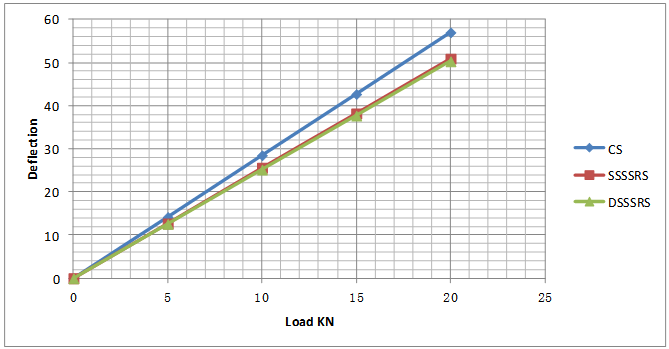• Figure 12. Load Deflection Curve for the Control and Improved Specimens